# Curve Shapes and Equations

In this worksheet, students must identify the equations of quadratic, cubic and hyperbolic curves.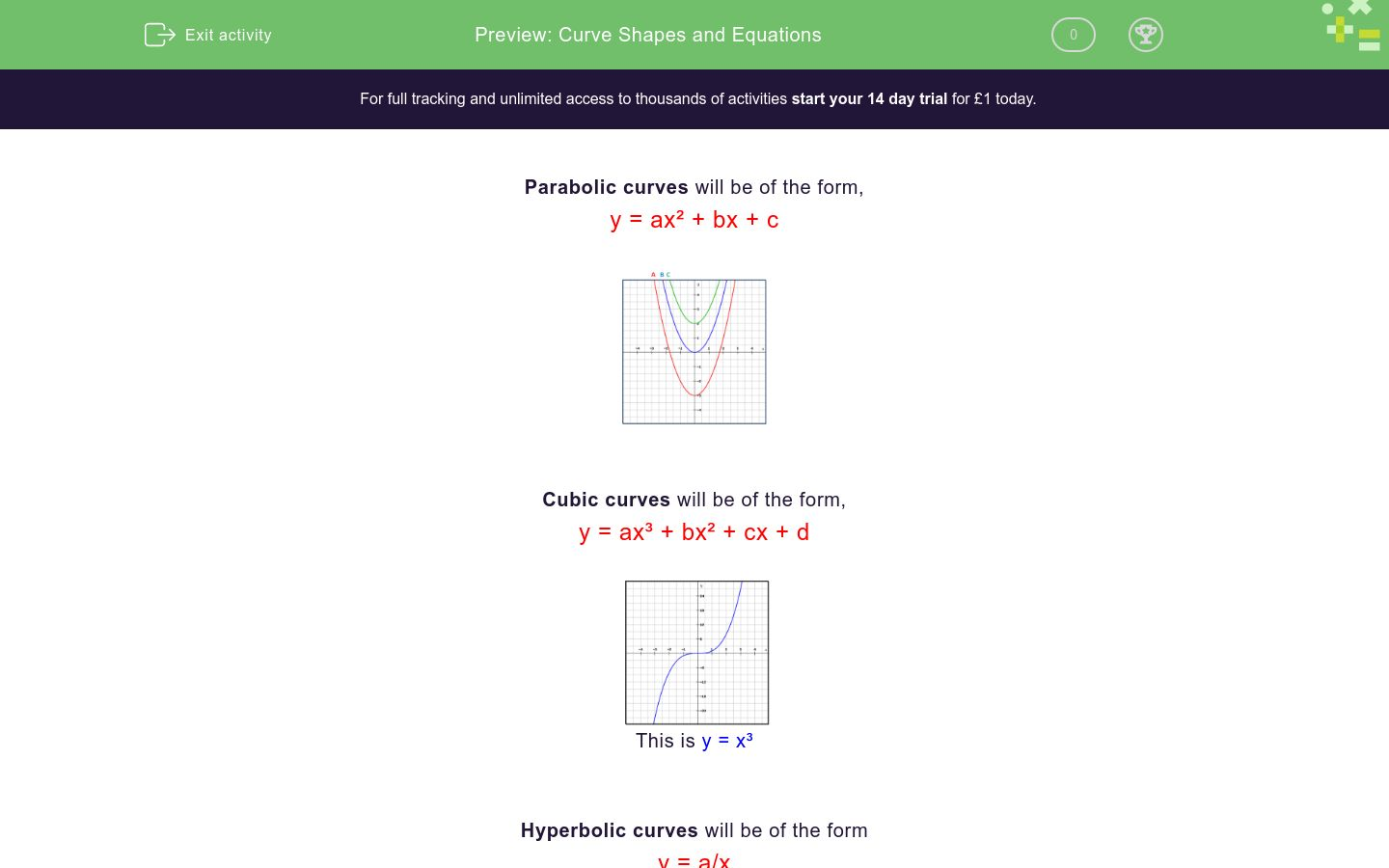Key stage:  KS 4

Curriculum topic:  Algebra

Difficulty level:### QUESTION 1 of 10

Parabolic curves will be of the form,

y = ax² + bx + c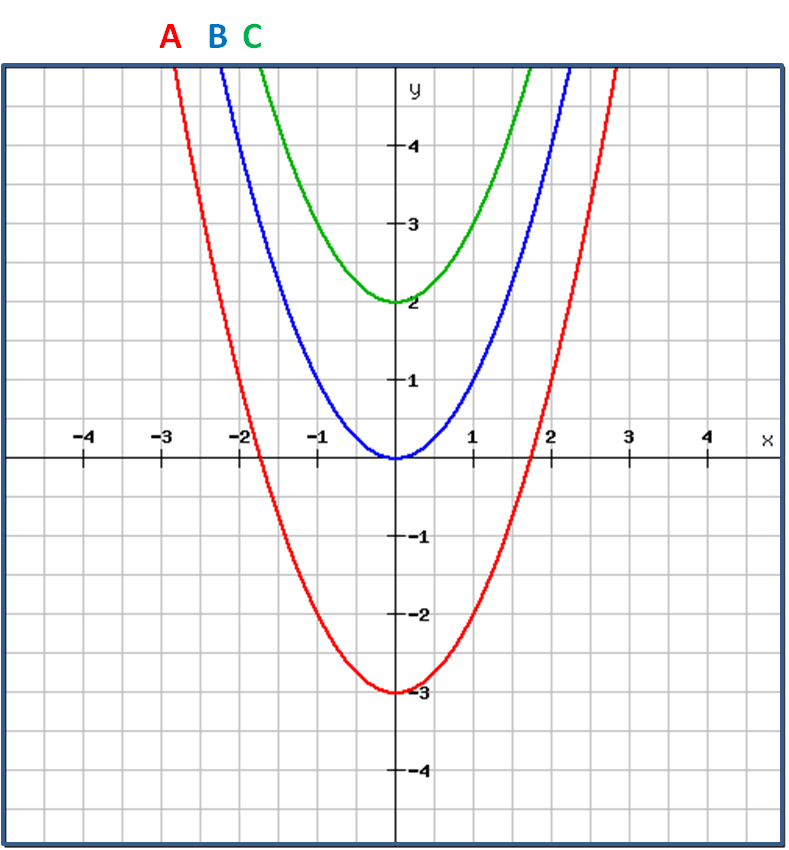Cubic curves will be of the form,

y = ax³ + bx² + cx + d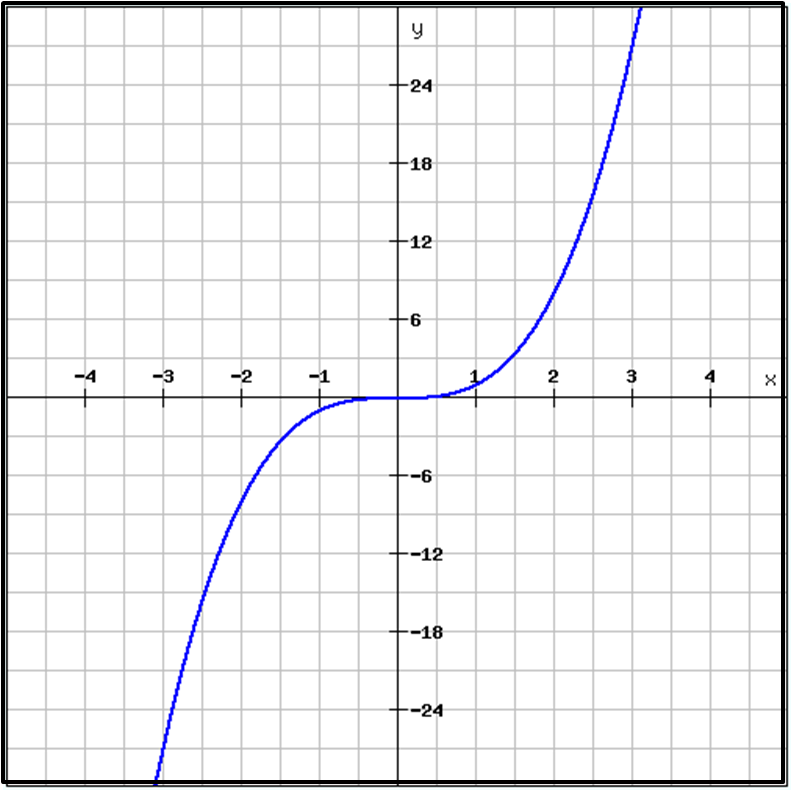This is y = x³

Hyperbolic curves will be of the form

y = a/x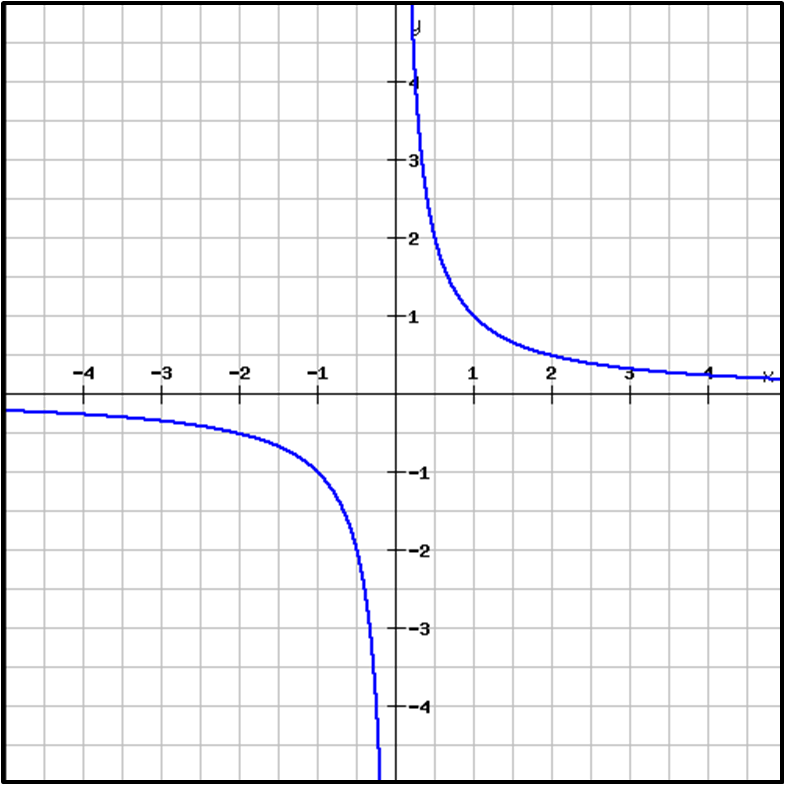This is y = 1/x

Note how the equation changes when we reflect a curve in the x-axis.This is y = x³               This is y = -x³This is y = 1/x             This is y = -1/x

Identify the correct equation of the curve shown below.y = -3x²

y = x³

y = -x²

y = -x³

y = 2x³

y = 1/x

y = -1/x

Identify the correct equation of the curve shown below.y = -3x²

y = x³

y = -x²

y = -x³

y = 2x³

y = 1/x

y = -1/x

Identify the correct equation of the curve shown below.y = -3x²

y = x³

y = -x²

y = -x³

y = 2x³

y = 1/x

y = -1/x

Identify the correct equation of the curve shown below.y = -3x²

y = x³

y = -x²

y = -x³

y = 2x³

y = 1/x

y = -1/x

Identify the correct equation of the curve shown below.y = -3x²

y = x³

y = -x²

y = -x³

y = 2x³

y = 1/x

y = -1/x

Identify the correct equation of the curve shown below.y = -3x²

y = x³

y = -x²

y = -x³

y = 2x³

y = 1/x

y = -1/x

Identify the correct equation of the curve shown below.y = -3x²

y = x³

y = -x²

y = -x³

y = 2x³

y = 1/x

y = -1/x

Identify the correct equation of the curve labelled A below.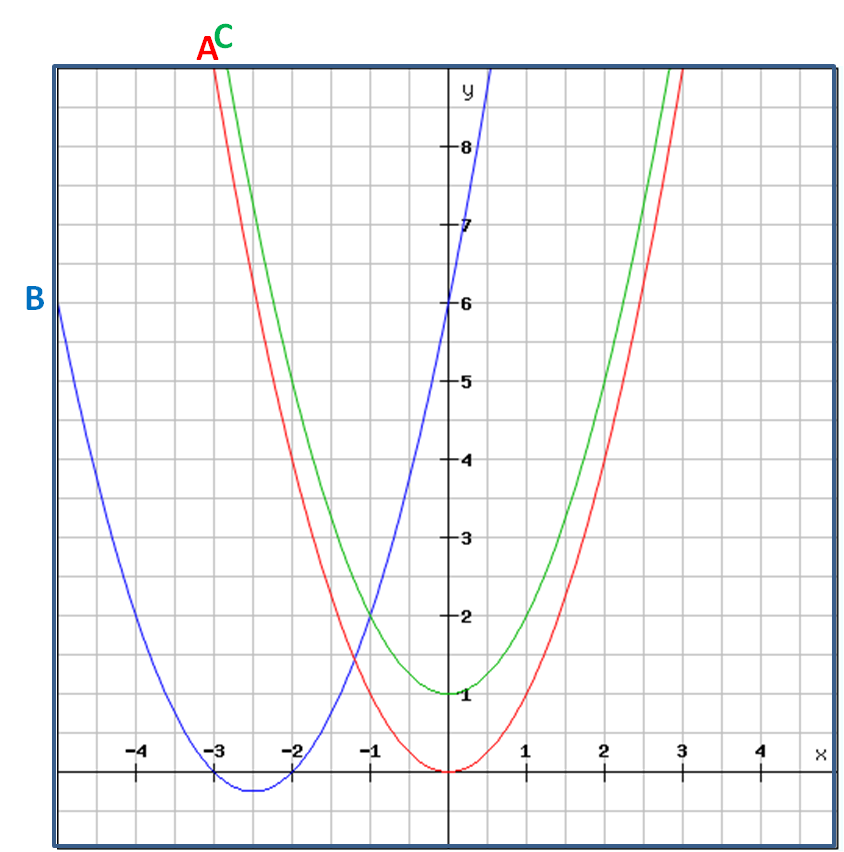y = (x + 1)²

y = (x + 2) (x + 3)

y = x²

y = (x - 1)²

y = x² + 1

Identify the correct equation of the curve labelled C below.y = (x + 1)²

y = (x + 2) (x + 3)

y = x²

y = (x - 1)²

y = x² + 1

Select all the possible correct equations for the curve labelled B below.y = x² - 5x - 6

y = (x + 2) (x + 3)

y = (x - 2) (x - 3)

y = (x + 3) (x + 2)

y = x² + 5x + 6

• Question 1

Identify the correct equation of the curve shown below.y = -x²
EDDIE SAYS
This is y = x² reflected in the x-axis.
• Question 2

Identify the correct equation of the curve shown below.y = x³
EDDIE SAYS
This is y = x³
The line passes through (0, 0), (1, 1), (2, 8) (-2, -8) etc.
• Question 3

Identify the correct equation of the curve shown below.y = 1/x
EDDIE SAYS
This is y = 1/x
The line passes through (1, 1), (2, ½) (-2, -½), (½, 2) etc.
• Question 4

Identify the correct equation of the curve shown below.y = -x³
EDDIE SAYS
This is y = -x³
The line passes through (0, 0), (1, -1), (2, -8) (-2, 8) etc.
• Question 5

Identify the correct equation of the curve shown below.y = -3x²
EDDIE SAYS
This is y = 3x² reflected in the x-axis.
• Question 6

Identify the correct equation of the curve shown below.y = -1/x
EDDIE SAYS
This is y = -1/x
The line passes through (1, -1), (2, -½) (-2, ½), (½, -2) etc.
• Question 7

Identify the correct equation of the curve shown below.y = 2x³
EDDIE SAYS
This is y = 2x³
The line passes through (0, 0), (1, 2), (2, 16) (-2, -16) etc.
• Question 8

Identify the correct equation of the curve labelled A below.y = x²
EDDIE SAYS
This is y = x²
The line passes through (0, 0), (1, 1), (2, 4) (-2, 4) etc.
• Question 9

Identify the correct equation of the curve labelled C below.y = x² + 1
EDDIE SAYS
This is y = x² shifted up 1 unit.
The line passes through (0, 0+1), (1, 1+1), (2, 4+1) (-2, 4+1) etc.
• Question 10

Select all the possible correct equations for the curve labelled B below.y = (x + 2) (x + 3)
y = (x + 3) (x + 2)
y = x² + 5x + 6
EDDIE SAYS
This is y = x² + 5x + 6.
The line passes through (-3, 0) and (-2, 0), so y = (x + 2)(x + 3).
---- OR ----

Sign up for a £1 trial so you can track and measure your child's progress on this activity.

### What is EdPlace?

We're your National Curriculum aligned online education content provider helping each child succeed in English, maths and science from year 1 to GCSE. With an EdPlace account you’ll be able to track and measure progress, helping each child achieve their best. We build confidence and attainment by personalising each child’s learning at a level that suits them.